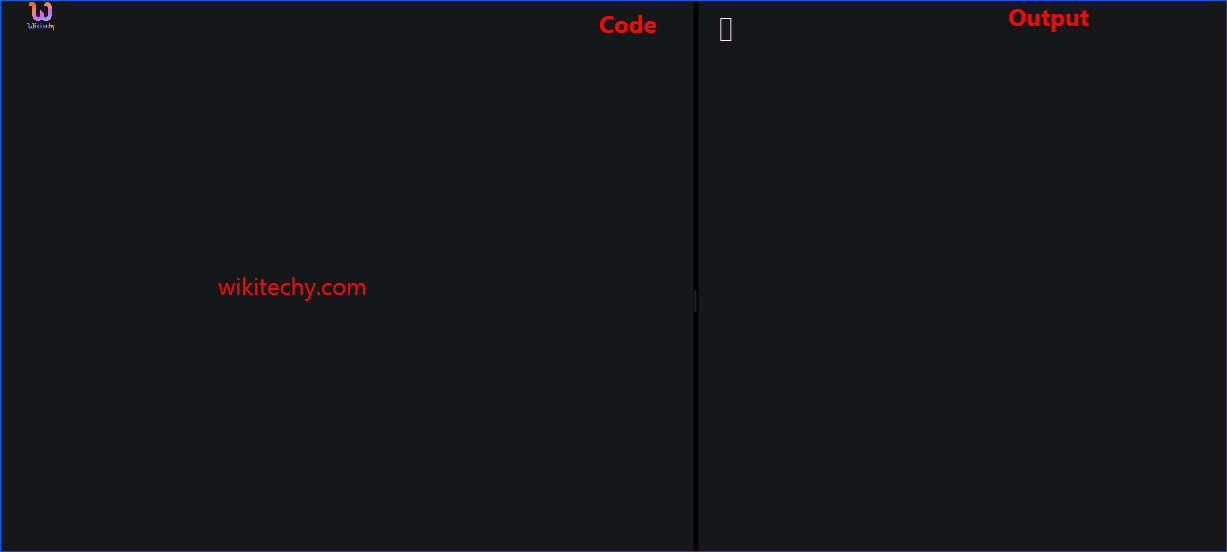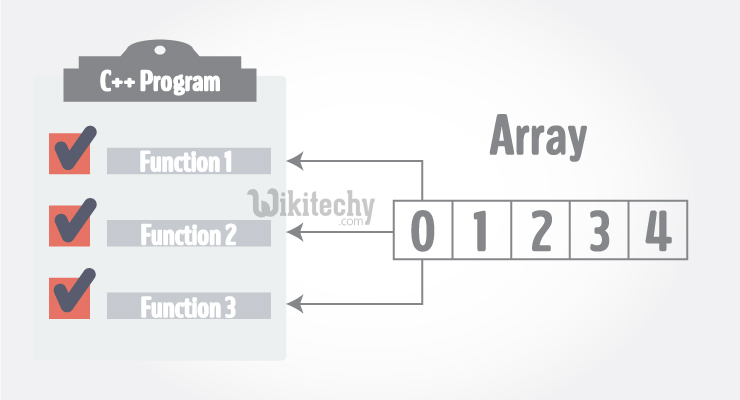# Passing 2d Array to Function C++ | Passing Array to a Function in C++ Programming - Learn C++ - C++ Tutorial - C++ programmingLearn c++ - c++ tutorial - passing-2d-array-to-function-c++ - c++ examples - c++ programs## Passing Array to a Function:

• C++ does not allow to pass an entire array as an argument to a function. However, You can pass a pointer to an array by specifying the array's name without an index.
• If you want to pass a single-dimension array as an argument in a function, you would have to declare function formal parameter in one of following three ways and all three declaration methods produce similar results because each tells the compiler that an integer pointer is going to be received.
• Arrays can be passed to a function as an argument. Consider this example to pass one-dimensional array to a function:

## Example 1: Passing One-dimensional Array to a Function

• C++ Program to display marks of 5 students by passing one-dimensional array to a function:

## Output:

• When an array is passed as an argument to a function, only the name of an array is used as argument.
• Also notice the difference while passing array as an argument rather than a variable.
• The argument marks in the above code represents the memory address of first element of array marks.
• And the formal argument int m in function declaration converts to int* m;. This pointer points to the same address pointed by the array marks.
• That's the reason, although the function is manipulated in the user-defined function with different array name m, the original array marks is manipulated.
• C++ handles passing an array to a function in this way to save memory and time.

## Passing Multidimensional Array to a Function:

• Multidimensional array can be passed in similar way as one-dimensional array. Consider this example to pass two dimensional array to a function:

## Example: Passing Multidimensional Array to a Function

• C++ Program to display the elements of two-dimensional array by passing it to a function.

## Output:

• In the above program, the multi-dimensional array num is passed to the function display().
• Inside, display() function, the array n (num) is traversed using a nested for loop.
• The program uses 2 for loops to iterate over the elements inside a 2-dimensional array. If it were a 3-dimensional array, you should use 3 for loops.
• Finally, all elements are printed onto the screen.

## Note:

• Multidimensional array with dimension more than 2 can be passed in similar way as two-dimensional array.